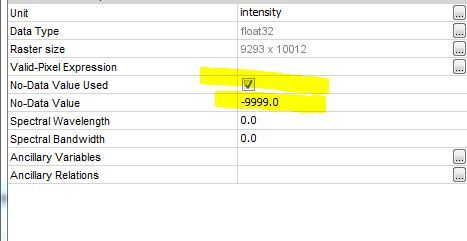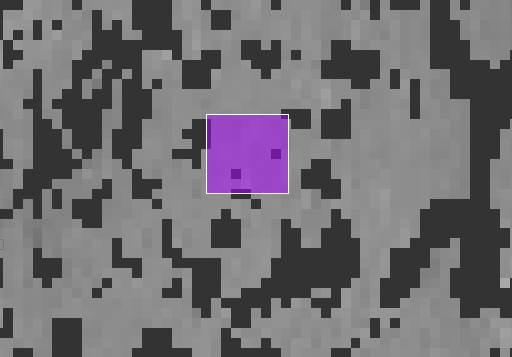NaN values in SNAP toolbox

I was using band math in SNAP toolbox for arithmetic operations. There are NaN values in final output. I tried to solve the equations using Python coding and it gives an output value as ‘1’. What is the problem.
I unchecked the band math tab, “Replace Nan and infinity results by”, even then the problem persist and gives the values as NaN.
Where am I wrong?

If you are using python, head to numpy and num_to_num().

What kind of data are you using? Maybe the NaN values are already in your input data. Maybe the input bands have a valid pixel expression or a no-data value. Check the properties of your input bands.
An other options is that you might need to delete the valid pixel expression of the resulting band.

1 Like

I have the similar problem. My data has two values of NaN and 0. I would like to replace NaN with 1 for instance. I am only interested in NaN pixels. In bandmath this is what I write: if myband== NaN then myband==1 else 0
But nothing really changes!
I have also tried one more time where “Replace Nan and infinity results” was checked and I put the value of “1” for. Again no change. Still I have NaN values!

Any suggestion!
Thanks

Check in the band properties what the no data value is for the band. Say for example the no data value is -999 and ‘use no data value’ is on then any -999 values show up a NaN.

1 Like

Thanks for your reply. The No-Data Value in properties was 0, and I’ve changed it to -9999. But still I get NaN as the pixel values on Sar image!

Even after this change, through the bandmath, I can not get the NaN mask where I say myband==NaN, it gives me a pure balck image everywhere with a value of 0!

I just need to work on areas with NaN value, but before bandmath I need to convert it to other values!

Thanks againTry in BandMaths: nan(myBand)

1 Like

It works now by doing so. Thanks a lot!I dont know why this time nan doesnt work, and still I get the value of NaN in a binary format!
Thanks

Mmmh?
Maybe check the valid pixel expression on the resulting band and remove the expression.
By default the input bands to the expression are used to define the valid pixel expression of the output.

1 Like

Valid data expression is blank. But now I think I know why that happened: After changing no data value to -9999, no data value should be remained Unchecked. Then the NaN value will be changed to 0.

Thanks again for your help.

Hello…How would be possible to change a value to ‘NaN’?

You need to create a new band and replace values by NaN.
Use the Band Maths for this.
As the expression, you can use something like:

BAND_NAME > 1.0 ? NaN : BAND_NAME

This will replace all values higher 1.0 with NaN in the band ‘BAND_NAME’

I have experienced some strange behaviour with this. I really struggled to remove a line of NaN between 2 S1 scenes that would not overlap.
What I did
untick the No-Data Value used in the property of the band Sigma0_VH

calculate the median 5x5 of the band

Use a first expression to produce the band Step1 Nonan
if nan(Sigma0_VH) then Sigma0_VH_median5 else Sigma0_VH

It would not replace directly the values by the ones of Sigma0_VH_median5 but produced 0s where there was NaN before
All the methods aforementioned would leave the stripe of NaN

Then I used this expression to finally get what I wanted at the seam between the 2 scenes.
Step1 Nonan == 0 ? Sigma0_VH_median5 : Step1 Nonan

Than a 3x3 median filter to remove the ugly edge (I actually wonder how the 5x5 filter is calculated around the NaN Stripe (1px wide) seems very sharp 3x3 would not work for some reason and leave the stripe

Certainly not the most beautiful way of achieving it but that is the only one I found out to work.
Hope it could help

Hello,

I have created a stack of four images derived from InSAR. I am looking at a ground displacement and I want to calculate some simple statistics (mean, max, min) for a given area in python using rasterstats library. The product has three bands:
band1. Displacement in LOS
band2.coherence map
band3.Absolute displacement in LOS

I loaded the band3 in python and wanted to calculate the mean value for the area inside the bbox as shown in figure below.
The values within the bbox range between -5 and 1. The MAX value is 1The dark pixels in the image are NaN values. There is an option in ‘rasterstats’ to do statistics ignoring the NaN values. When i did some statistics, the MAX value of NaN pixels were replaced by the value -50.44. So, all NaN pixels had the same value of -50.44.

So, i did a little bit of investigation playing around with SNAP to see why NaN values have been replaced by the value -50.44. I removed the Valid-Pixel Expression of the the band and left it blank and I got the following image.

Surprisingly, All the NaN values (the black pixels of the previous image) are now replaced by the value -50.44 (all the white pixels), that’s why the statistic calculations in python were all messed up.

Is there any explanation on why this specific value is assigned to NaN pixels?
What I have noticed is that the values of band1 range between -52 and -55. Its like, it got this value from the band one and assigned it in the band 3 as NaN value.
Same thing happened with a stack of 17 images. The band1 of the product with the stack of 17 images has values between -210 and -212. When trying to do statistics using the band 3(which contains the absolute displacement values), the NaN values are replaced by the value of -212.
It is bit mystery to me.

Finally, the last experiment i did, it was to download a random Ground Range Detected SAR image and do the same. In this case, when I removed the Valid-Pixel Expression of the the band and left it blank, the NaN values around image were replaced to zero but the NaN values inside the image remained as NaN values. This is what i expected to happen.
This is not he case for the InSAR stack though.

I do not know if anyone can give me an explanation on that
Thank you

Actually, I have just resolved the problem of my previous post. The expression i have used is the following:
band3==0 ? NaN : band3
Replace all zeros to NaN values for the band3 (which is the displacement map in LOS). Now, this can be exported to python and do statistics ignoring the NaN values.

3 Likes

Maybe @cwong or @timmoorhouse can explain why the invalid values (-50.44) are as they are. This is somewhere done in the processing.

Great, that you could find a solution.

@lveci I have a mask with NaNs and 1s. I’m interested in turning all NaN’s to 1 and all 1s to zero. Can you please help.

regards

Band Maths:

IF raster== NaN THEN 1 ELSE 0

@ABraun Using your help; "1"s are replaced with zeros. But NaNs aren’t turning to 1s. Any two cents on this? Thanks!